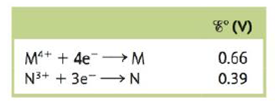# Consider a galvanic cell based on the following theoretical half-reactions: What is the value of ∆ G ° and K for this cell?### Chemistry: An Atoms First Approach

2nd Edition
Steven S. Zumdahl + 1 other
Publisher: Cengage Learning
ISBN: 9781305079243

#### Solutions

Chapter
Section### Chemistry: An Atoms First Approach

2nd Edition
Steven S. Zumdahl + 1 other
Publisher: Cengage Learning
ISBN: 9781305079243
Chapter 17, Problem 136CWP
Textbook Problem
5 views

## Consider a galvanic cell based on the following theoretical half-reactions:What is the value of ∆G° and K for this cell?

Interpretation Introduction

Interpretation:

The value of ΔG° and K for the given cell is to be calculated.

Concept introduction:

The species with higher oxidation potential undergoes reduction reaction at the cathode, while the species with lower reduction potential value undergoes oxidation at the anode.

The relationship between Gibbs free energy change and cell potential is given by the formula,

ΔG°=nFE°cell

The relationship between Gibbs free energy change and equilibrium constant is given by the formula,

ΔG°=RTlnK

To determine:

The value of ΔG° and K for the given cell is to be calculated.

The value of ΔG° is -312.611kJ_ .

### Explanation of Solution

The reaction taking place at the cathode is,

M4++4eME°red=0.66V

The reaction taking place at the anode is,

NN3++3eE°OX=0.39V

Multiply reduction half-reaction with a coefficient of 3 and oxidation half-reaction with a coefficient of 4 and then add both the reactions as,

3M4++12e3M4N4N3++12e

The final equation is,

3M4++4N3M+4N3+

The value of E°cell is given as,

E°cell=E°ox+E°red

Where,

• E°ox is the oxidation potential of the electrode.
• E°red is the reduction potential of the electrode.

Substitute the values of E°ox and E°red in the above equation as,

E°cell=E°ox+E°red=0.39V+0.66V=0.27V

The reaction involves the transfer of 12 moles of electrons.

The relationship between Gibbs free energy change and cell potential is given by the formula,

ΔG°=nFE°cell

Where,

• ΔG° is the Gibbs free energy change at the standard conditions.
• n is the number of moles of electrons that are involved in the reaction.
• F is the Faraday’s constant (96,485C/molee)
• E° is the cell potential at the standard conditions.

Substitute the value of n , F and E° in the above equation as,

ΔG°=nFE°cell=12e×96485Cmolee×0

### Still sussing out bartleby?

Check out a sample textbook solution.

See a sample solution

#### The Solution to Your Study Problems

Bartleby provides explanations to thousands of textbook problems written by our experts, many with advanced degrees!

Get Started

Find more solutions based on key concepts

Fundamentals of Physical Geography

Methylmercuryis a toxic industrial pollutant that is easily destroyed by cooking. T F

Nutrition: Concepts and Controversies - Standalone book (MindTap Course List)

What are the base-pairing rules for DNA? a. AG, TC b. AT, GC c. AC, TG d. AA, GG, CC, TT

Biology: The Unity and Diversity of Life (MindTap Course List)

How can errors in the cell cycle lead to cancer in humans?

Human Heredity: Principles and Issues (MindTap Course List)

To which spectral classes do the stars in Problem 4 belong?

Horizons: Exploring the Universe (MindTap Course List)

Match the terms and definitions.

Human Biology (MindTap Course List)

25. Name each compound:

Chemistry In Focus

Besides the gravitational force, a 2.80-kg object is .subjected to one other constant force. The object starts ...

Physics for Scientists and Engineers, Technology Update (No access codes included)

Is most of Earths water in the ocean?

Oceanography: An Invitation To Marine Science, Loose-leaf Versin